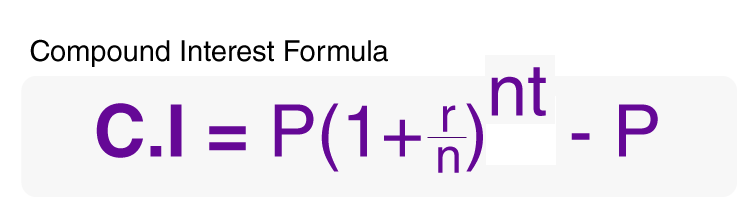# Compound Interest Formula

Compound interest formula is mentioned and explained here along with a solved example. To recall, compound interest can be defined as “An interest on interest to the principal sum of a loan or deposit.” In simple words, the compound interest is the interest that adds back to the principal sum, so that interest is earned during the next compounding period. Here, we will discuss maths compound interest questions with solutions and formulas in detail.## Compound Interest Formula

The formula for the Compound Interest is,

$$\begin{array}{l}Compound\;Interest\,=\,P(1+\frac{r}{n})^{nt}\,-\,P\end{array}$$

This is the total compound interest which is just the interest generated minus the principal amount. For the total accumulated wealth (or amount), the formula is given as:

$$\begin{array}{l}A\,=\,P(1+\frac{r}{n})^{nt}\end{array}$$

### Notations in Compound Interest Formula:

 C.I. Compound Interest P Principal Amount A Total Accumulated Amount r Rate of Interest n Compounding Frequency Per Annum t Time (in Years)

Note: If the compounding frequency per annum is 1 i.e. if the interest is compounded annually, the compound interest formula is given as:

$$\begin{array}{l}C.I.\,=\,P(1+\frac{r}{100})^{t}\,-\,P\end{array}$$

Also Check: Simple Interest Formula

### Maths Compound Interest Questions with solutions

Question:

A sum of Rs. 50,000 is borrowed and the rate of interest is 10% per annum. What is the compound interest for 5 years?

Solution:

From the formula for compound interest, we know,

C.I = P(1+R⁄100)t– P

Here, P = 50,000 ; R = 10% ; T = 5 years ; C.I=?

So, Compound Interest will be-

C.I. = 50000(1+10⁄100)5 – 50000 = 80525.50 – 50000

So, compound interest will be Rs. 30525.50 while the total accumulated wealth (A) will be Rs. 80525.50 at the end of 5 years with an interest rate of 10% per annum.